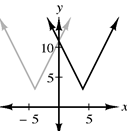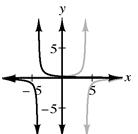### Home > INT3 > Chapter 4 > Lesson 4.2.2 > Problem4-58

4-58.

For each of the following functions, sketch the graph of the original and of $y=f(-x)$.

1. $f(x)=2|x-4|+3$

On a graph, where are the positive $x$-values in relationship to the negative $x$-values?

$f(−x)=2|−x−4|+3$
$f(−x)=2|−(x+4)|+3$
$f(−x)=2|x+4|+3$1. $f\left(x\right)=\frac{1}{x+4}$

See part (a).1. Is each of these functions odd, even, or neither? Justify your answer.

Are either of these graphs symmetrical over the $y$-axis (even) or a rotation about the origin (odd)?